# Water Images

When an object is placed near water source like river, pond or water tub etc, then the image formed in the water is called water image of the object.

Water Image is the reflection of an object into water. It is the vertically inverted image obtained by turning the object upside down. The water image of the figure looks like the mirror image of the figure when the mirror is placed horizontally at the bottom of the question figure.

From the below figure, it is clear that the water image is an inverted form of a real object in which LHS (Left Hand Side) and RHS (Right Hand Side) remains unchanged but the top and bottom of the object gets interchanged i.e. top becomes bottom and bottom becomes top.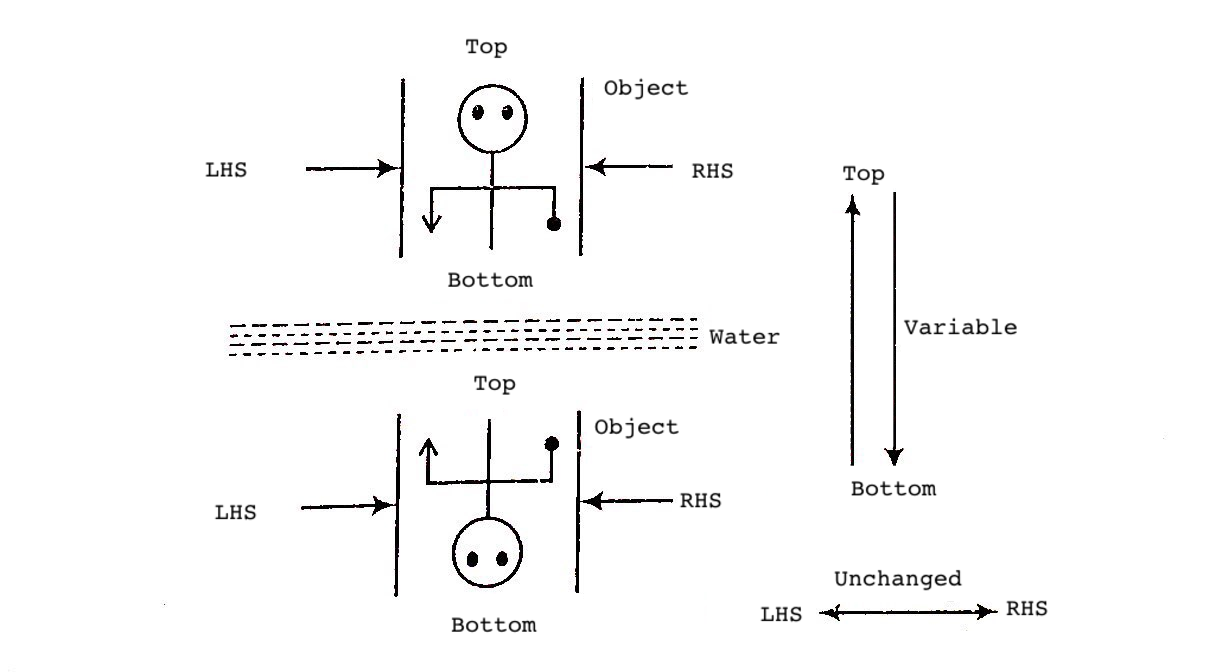Different types of questions covered in this section are as follows

1. Water Image of Figure/Symbol/Sign

2. Water Image of Letters and Numbers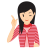MIND IT !

In questions based on water image, the candidate is required to determine how the image of object would look like in water when it is placed near water source.

Mostly the water image of a figure is different from the original figure which is because of the dissimilarity in the upper and lower half of the figure. This can be better understood with the help of example illustrated below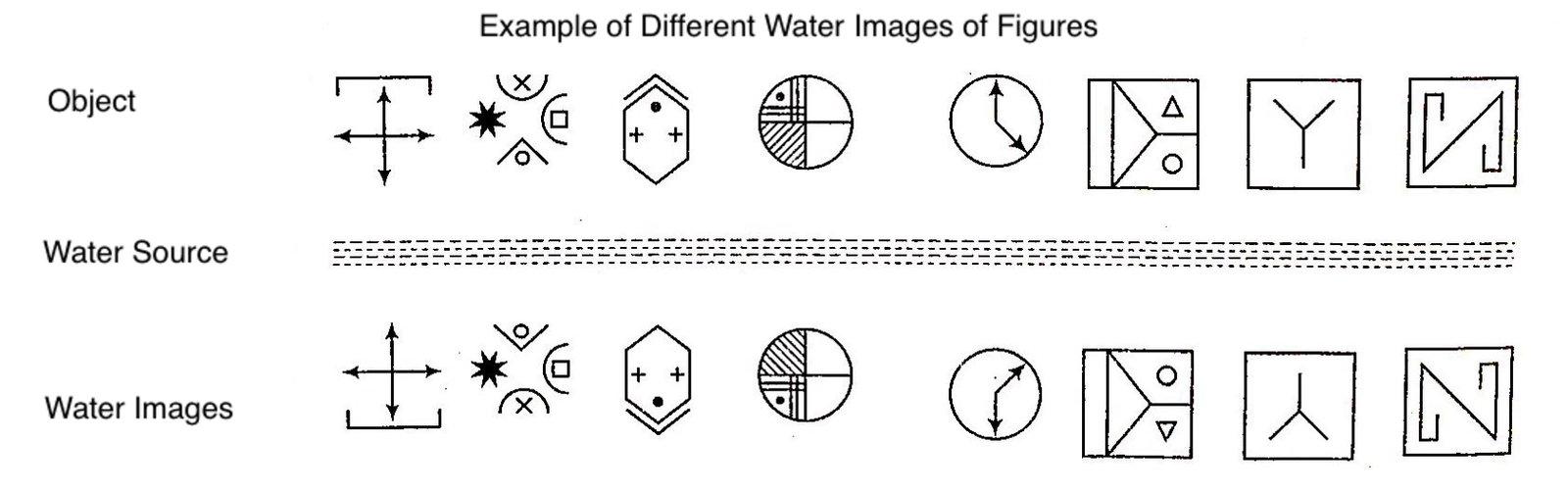Sometimes it happens that water image formed is identical to the original figure. This is the case when upper half of the figure is similar to the lower half of the figure but in opposite direction. This can be better understood with the help of identical water images of figures which are given below

Example of Identical Water Images of Figures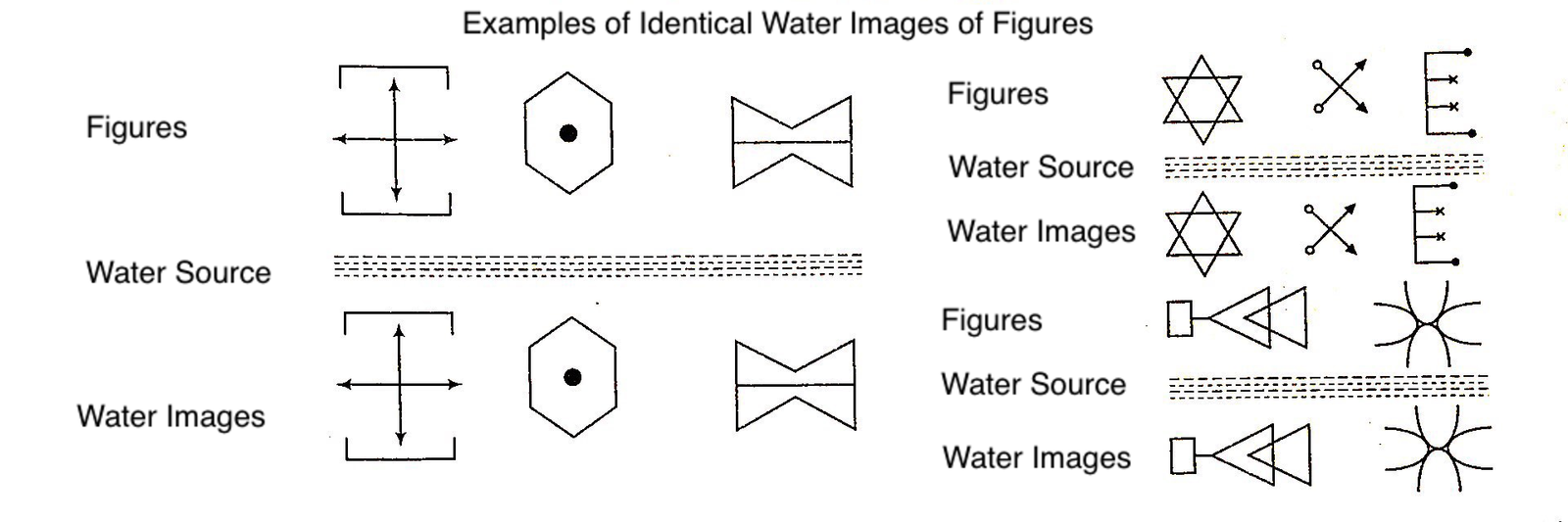From the above examples, it is clear that in the water image, (LHS) and (RHS) remain unchanged while upper and lower parts get interchanged, means top becomes bottom and bottom becomes top.## Types of Questions

There are two types of questions based on water images, which can be asked in various competitive examinations

## Type #1 : Water Image of Figure/Symbol/Sign

In this type of questions, candidates are asked to find the water image of symbol, signs or figure which consists of both symbol and signs or any other pattern. The following examples will give a better idea of the questions asked in exams.

Directions (Example Nos. 1-4) Find the water image of the object given in the question figure denoted by (A), out of the figures given in the answer choices, (a), (b), (c) and (d).

Example 1: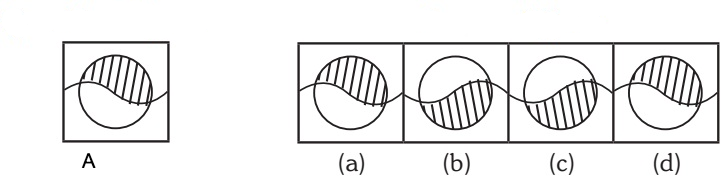Solution: The figure in option (b) represent the correct water image of figure (A). Upper part will go down in opposite direction in water image.

Example 2: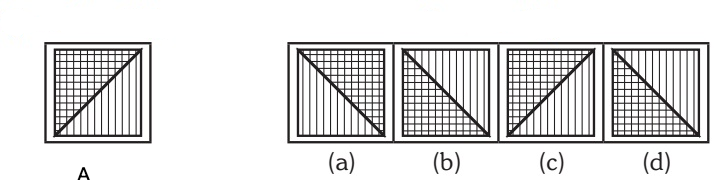Solution: The figure in option (d) represent the correct water image of figure (A). Upper part will go down in opposite direction in water image.

Example 3: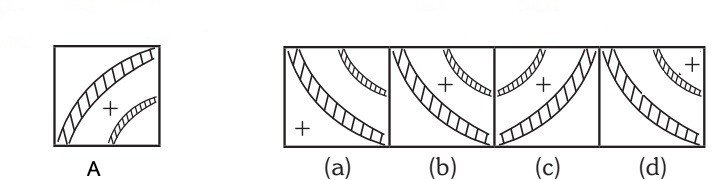Solution: The figure in option (b) represent the correct water image of figure (A). Upper part will go down in opposite direction in water image.

Example 4: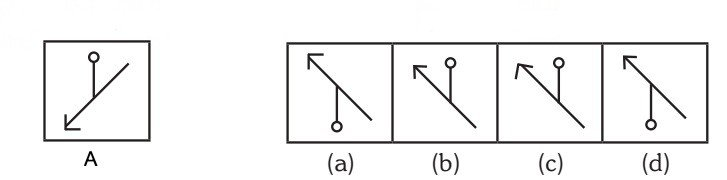Solution: The figure in option (a) represent the correct water image of figure (A). Upper part will go down in opposite direction in water image.

## Type #2 : Water Image of Letters and Numbers

The candidates are required to learn how the letters and numbers get reflected in water and follow the same to solve the problems based on water image of letters and numbers.

Water Image of Capital Letters :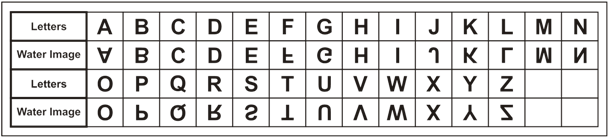The letters which have water images identical to the original letter are - C, D, E, H, I, O, X

Water Image of Small Letters :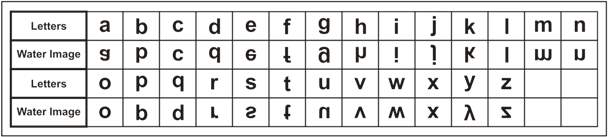The letters which have water images identical to the original letter are - c, i, o, x

Water Image of Digits :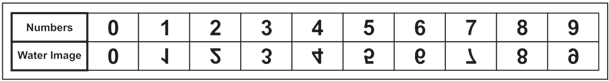Number 0, 3 and 8 have water images similar to the original numbers.

The following example will give a better idea about the types of questions asked in exams

Directions (Example Nos. 5-7) In each of the following questions, find the water image of the set of letters and/or digits given in the question figure out of the four answer choices (1), (2), (3) and (4).

Example 5: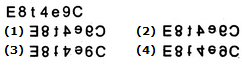Solution: Reflection of the given group of letters and numbers would be seen in the water as : Option 4

Example 6: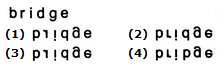Solution: The given word's reflection would be seen in the water as : Option 2

Example 7: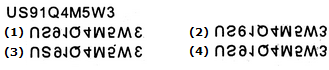Solution: Reflection of the given group of letters and numbers would be seen in the water as : Option 4

## TIPS on cracking Non Verbal Reasoning Questions on Water ImagesFew Important things to Remember

Time is a very important thing in every exams. You should complete your exam within the time frame. But in a competitive exam, question are for testing your calculation ability within a given time frame. They tests, how quickly a student can solve a question paper. This is the reason so many students couldn’t finish their paper within given time. But if you need to solve your government or bank or any other question paper quickly then you should use tips of reasoning and Water Images which will help you to solve government, bank and other exam papers quickly.

Tip# : Water Image of a figure has all parts of the figure vertically inverted

Thus, here:

1.    The water images of alphabets B, C, D, E, H, I, K, O, X, and of digits 0, 8, remain the same as the original.

2.    The water image of a sequence of words and/or numbers is the water image of each, written in the same order.

3.    The figure should remain the same vertically.

Question: Choose the alternative which closely resembles the water-image of the given combination.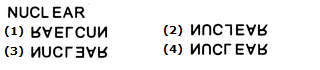Solution:

Option (1) can be eliminated since it is laterally inverted as well. Again, in (2), L and in (3), E is laterally inverted as well. Only (4) is the correct water image of (X).